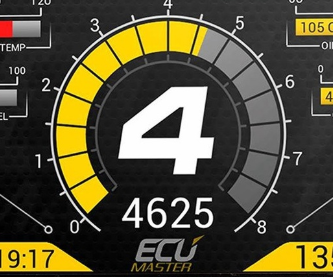# Drawing a circle with different increments

i am trying to make a circle around this text label, but it takes a while to draw it all. how can i use code to draw a circle with a set amount of rotation increment?

4 Likes

Can you please explain how you are currently drawing the circle, and share your code? We can’t help otherwise.

Or do you mean you’re doing it by hand and want a better way?

doing it by hand, i want a more efficient way to do this

The easiest way would be to use the negate tool to cut a cylinder-shaped hole in a cylinder. In which case, this thread belongs in Building SupportYou could also make it out of several parts with scripting, but it doesn’t seem necessary

it really has to do with gui elements, so that is why i was trying to make a script that clones the gui element and puts it next in the circle

that is the basis of what i was trying to do

Are you trying to make a loading icon? Like a spinner which fills up from 0% to 100%

Like this?

no, im trying to make the outline of a gauge. im trying to use frames with different angles to make an almost circle.

here is what i am gunning for:i have a basic idea of what to do for this, so its just the ui elements is where it will take a while without a bit of code

Ah gotcha. As a suggestion, it helps to put what you’re trying to do in your first post, rather than asking about the way you’ve decided to approach the problemAnyways, I would suggest using an image for something like that. It’s gonna be easier. But, if you’re determined…

``````local function CreateArc(startAngle, endAngle, segments, innerRadius, thickness)
thickness = thickness or 4

local frame = Instance.new("Frame")
frame.Name = "Arc"

local sideLen = 2 * math.tan(theta) * innerRadius

for i = 0, segments - 1 do
local angle = startRad + theta + (endRad) * i / (segments)
local x = math.cos(angle) * (innerRadius - thickness / 2)
local y = math.sin(angle) * (innerRadius - thickness / 2)

local f = Instance.new("Frame")
f.AnchorPoint = Vector2.new(0.5, 0.5)
f.Size = UDim2.fromOffset(sideLen, thickness)
f.Position = UDim2.fromOffset(x, y)
f.Rotation = math.deg(angle) + 90
f.BorderSizePixel = 0
f.Parent = frame
end

return frame
end

local frame = CreateArc(0, 270, 20, 200, 30)
frame.Position = UDim2.fromScale(0.5, 0.5)
frame.Parent = script.Parent
``````
2 Likes

Would this work? It would be better than using 100s of gui objects, just because 1 image label is less memory usage than 100+ frames, plus you can add more artwork easier than you could with just frames.
If you’d rather have a frame based circle, you can do it in the command line with this code:

``````local numberOfParts = 150
local BaseGui = workspace.Frame --path to whatever you want to make the frame with
local fullCircle = 2 * math.pi
local parts = {}

local x = math.cos(angle) * radius
local z = math.sin(angle) * radius
return x, z
end

for _ = 1, numberOfParts*(Radius/10) do
table.insert(parts, BaseGui:Clone())
end

for i, GUIItem in pairs(parts) do
local x, y = getXAndYPositions(i * (fullCircle / #parts), Radius)
local Angle = math.atan2( - x,  - y)
local ConvertedAngle = ((Angle / math.pi) * 180)
GUIItem.Rotation = 0 - ConvertedAngle
GUIItem.Position = UDim2.new(0,x,0,y)
GUIItem.Parent = Base
end
end

CreateCircle(game.StarterGui.ScreenGui.Frame, 50)
warn("Finished!")
``````

Note this circle will be created to the left, so you’ll likely need to move it. I simply used a {0,5}{0,5} size frame for the base frame, and a default frame as the base. It lagged quite a bit more than a single image label when I was moving it, but if this is what you would like, hope it helps.

6 Likes

this code is probably what ieas looking for, thabk you. the way im using the gui objects is for a working gauge so i need a lot of them to make it work.

i assume number of parts is how many gui objects will be created

1 Like